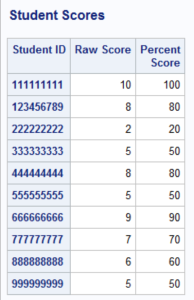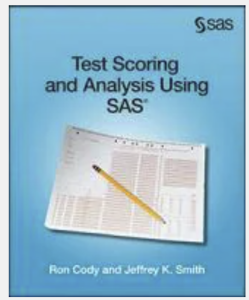If you have ever needed to score a multiple-choice test, this blog is for you. Even if you are not planning to score a test, the techniques used in this example are useful for many other programming tasks. The example I am going to present assumes the answer key and student answers are in a single file. A sample file for scoring is shown below:

Student IDs are in columns 1–9. (The answer key uses all zeros, but the program would work equally well if you left these columns blank.) The student answers are in columns 11–20. The "trick" is to read the answer key and place the correct answers in the variables Key1–Key10.  You can do this by writing a conditional INPUT statement that uses the internal SAS variable _N_ to let you know when you are reading the first observation. Let's take a look at the program and annotate it.

```data Score; infile 'c:\books\Test Soring\Sample_Test.txt' pad; ❶ array Ans \$ 1 Ans1-Ans10; ***student answers; ❷ array Key \$ 1 Key1-Key10; ***answer key; array Score Score1-Score10; ***score array 1=right,0=wrong; retain Key1-Key10; ❸   if _n_ = 1 then input @11 (Key1-Key10)(\$1.); ❹ input @1 ID \$9. @11 (Ans1-Ans10)(\$1.);   do Item=1 to 10; Score[Item] = Key[Item] eq Ans[Item]; ❺ end;   Raw=sum(of Score1-Score10); ❻ Percent=100*Raw / 10; keep Ans1-Ans10 ID Raw Percent; label ID = 'Student ID' Raw = 'Raw Score' Percent = 'Percent Score'; run;   proc sort data=Score; ❼ by ID; run;   title "Student Scores"; proc print data=Score label; ❽ id ID; var Raw Percent; run;```

❶ The data file is located in the C:\Books folder. The PAD option is useful if there are lines of data that contain fewer than 10 answers—it is an instruction to PAD each line with blanks up to the defined record length.

❷ You set up three arrays: Ans holds the student answers, Key holds the answer key, and Score is a variable that indicates if the answer is correct or not (1=correct, 0=incorrect).

❸ Because you are reading the Key values from raw data, you need to RETAIN these values so that SAS does not set them to a missing value on subsequent iterations of the DATA step.

❹ When _N_ is equal to 1, you read the variables Key1–Key10.

❺ This is an interesting statement. The logical expression Key[Item] eq Ans[Item] will be 1 if the student has the correct answer and 0 otherwise. This value is then assigned to the variable Score[Item].

❻ The raw score (the number of items answered correctly) is the sum of the variables Score1–Score10.

❼ For a more logical report, you sort the observations in ID order.

❽ You use PROC PRINT (or PROC REPORT if you prefer) to list the data. The PROC PRINT option LABEL causes the procedure to use variable labels (if they have labels—and they do) instead of the default use of variable names as column headings. By the way, the default behavior for PROC REPORT is to use variable labels.

Below is the output from this program.If you would like to know more about test scoring, including how to score tests with multiple versions or how to perform item analysis, please see my book Test Scoring and Item Analysis Using SAS (used copies are available on Amazon). As usual, comments or corrections are welcome. You may email me directly at Ron.Cody@gmail.com.Share